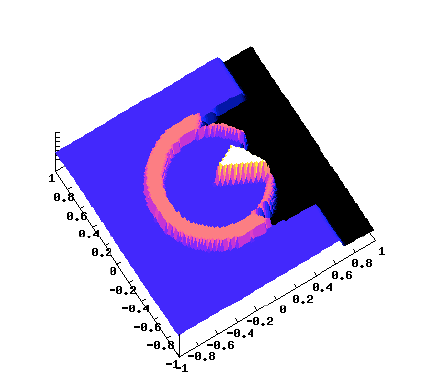# Coupling techniques for nonlinear hyperbolic equations. IV. Well-balanced schemes for scalar multi-dimensional and multi-component laws### Résumé

This series of papers is devoted to the formulation and the approximation of coupling problems for nonlinear hyperbolic equations. The coupling across an interface in the physical space is formulated in terms of an augmented system of partial differential equations. In an earlier work, this strategy allowed us to develop a regularization method based on a thick interface model in one space variable for coupling scalar equations. In the present paper, we significantly extend this framework and, in addition, encompass equations in several space variables. This new formulation includes the coupling of several distinct scalar conservation laws and allows for a possible covering in space. Our main contributions are, on one hand, the design and analysis of a well-balanced finite volume method on general triangulations and, on the other hand, a proof of convergence of this method toward entropy solutions, extending Coquel, Cockburn, and LeFloch’s theory (restricted to a single conservation law without coupling). The core of our analysis is, first, the derivation of entropy inequalities as well as a discrete entropy dissipation estimate and, second, a proof of convergence toward the entropy solution of the coupling problem.

Type
Publication
Math. Comp.